小试SVG之新手小白入门教程

更新时间：2019年01月08日 10:46:58   作者：王大拿我要评论

svg(Scalable Vector Graphics)是一种基于XML语法的图像格式，全称是可缩放矢量图，其它图像格式都是基于像素处理的，SVG则是属于对图像的形状描述，所以它本质上是文本文件，体积较小，且不管放大多少倍都不会失真.SVG是面向图形，HTML时面向文本。

SVG可以写在一个独立的文件中，然后用img, object, embed, iframe等标签插入网页

```<img src="circle.svg">
<object id="object" data="circle.svg" type="image/svg+xml"></object>
<embed id="embed" src="icon.svg" type="image/svg+xml">
<iframe id="iframe" src="icon.svg"></iframe>```

SVG文件可以转为base64编码，然后作为Data URI写入网页

`<img src="data:image/svg+xml;base64,[data]" />`

SVG书写的注意点

• SVG的元素和属性必须按照标准格式来写，因为XML是确认大小写的
• SVG里的属性值必须用引号引起来，就算是数值也必须这么做
• SVG图像的默认大小是300像素（宽）x 150像素（高）
• 后面的元素会渲染在前面元素之上

SVG的所有元素

SVG的所有元素

SVG的所有属性

SVG的所有属性path

`<rect d="M10 10" />'`

```<path d="M20,20 L40 40 M60 60 L80 80" fill="none" stroke="blue" stroke-width="5"/>

<path d="M20,20 L40 40 m60 60 L80 80" fill="none" stroke="blue" stroke-width="5"/>```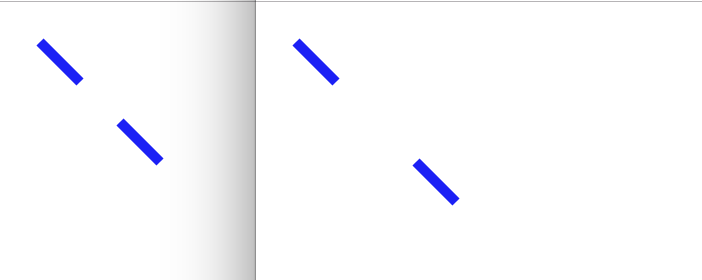• L：L指令会拿到两个参数，x坐标和y坐标，然后从当前位置到指定参数坐标位置来绘制线段
• H：H其实是horizontal的缩写，意为绘制出水平方向的线段，因为方向已确定，所以只需一个参数就能完成线段的绘制
• V：同H同理，只不过表示垂直方向（vertical）的线段绘制

step1

`<path d="M10 10 H 90" fill="none" stroke="blue"/>`step2

`<path d="M10 10 H 90 V 90" fill="none" stroke="blue"/>`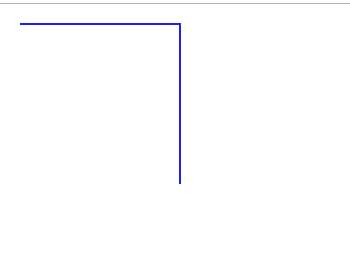step3

`<path d="M10 10 H 90 V 90 H 10" fill="none" stroke="blue"/>`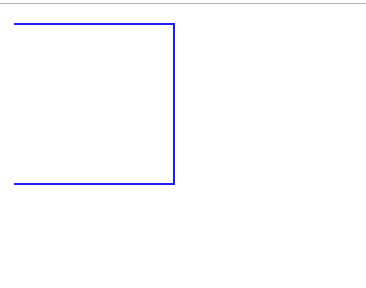step4

`<path d="M10 10 H 90 V 90 H 10 V 10" fill="none" stroke="blue"/>`Z：该指令的作用是从当前位置向起始点画出一条线段，它一般都被放置在一连串节点的末尾，并且不区分大小写。可以理解为”闭环“指令

`<path d="M10 10 H 90 V 90 H 10 Z" fill="none" stroke="blue"/>`

`<path d="M10 10 h 80 v 80 h -80 Z" fill="transparent" stroke="blue"/>`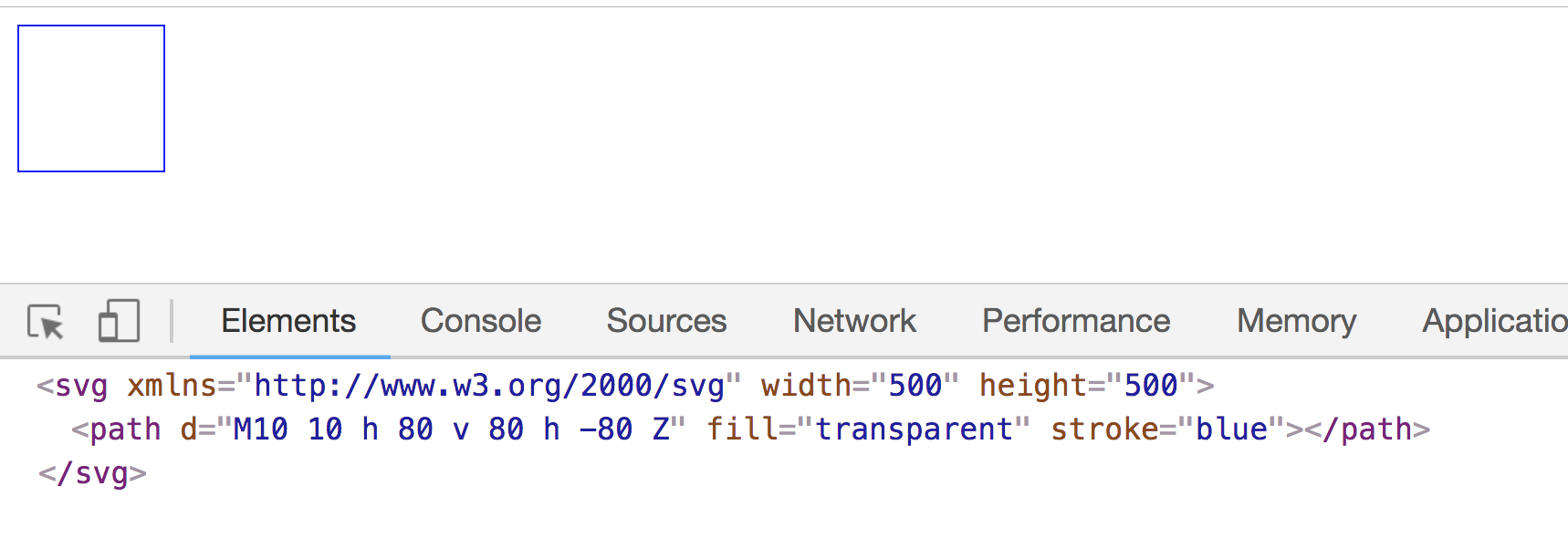C：该指令用于创建一个三次贝塞尔曲线，需指定三组参数

`<path d="M10 10 C 20 20, 40 20, 50 10" stroke="black" fill="transparent"/>`

MDN上有多组曲线的对比示例。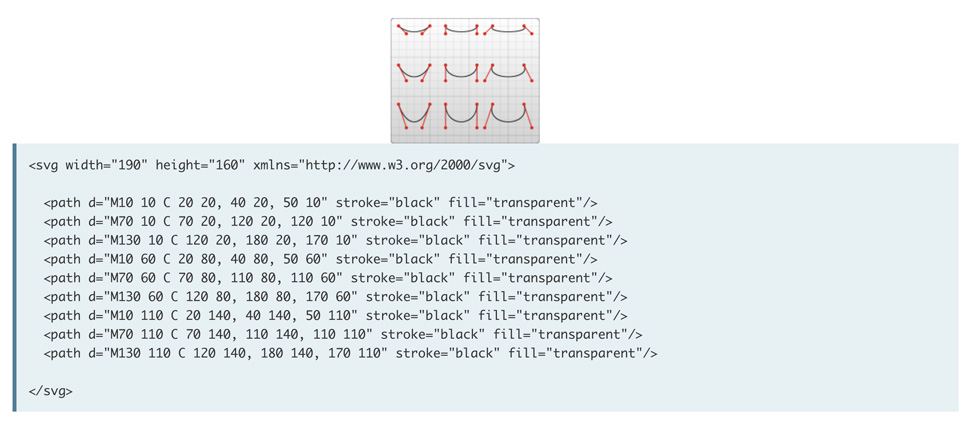`<path d="M10 10 C 10 10, 40 10, 50 10" stroke="black" fill="transparent"/>`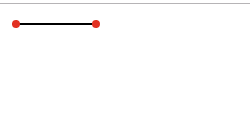• S指令跟在C或者另一个S指令之后：那S指令的开始控制节点就是基于前一个控制节点的对称点，并且S指令指定的第一组节点是结束控制节点
• 单独的S指令：两个控制节点会被设置为同一个点

``` <svg
xmlns="http://www.w3.org/2000/svg"
width="190"
height="160"
>
<path d="M10 80 C 40 10, 65 10, 95 80 S 150 150, 180 80" stroke="black" fill="transparent"/>

<circle cx="10" cy="80" r="2" fill="red"/>
<circle cx="95" cy="80" r="2" fill="red"/>
<circle cx="180" cy="80" r="2" fill="red"/>
<circle cx="150" cy="150" r="2" fill="red"/>
</svg>```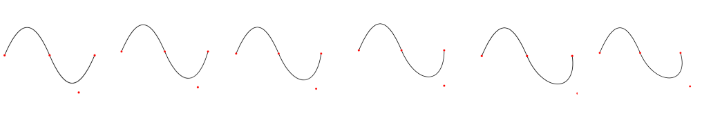```<svg
xmlns="http://www.w3.org/2000/svg"
width="300"
height="300"
>
<path d="M10 80 S 95 150, 180 80" stroke="black" fill="transparent"/>

<circle cx="10" cy="80" r="2" fill="red"/>
<circle cx="95" cy="150" r="2" fill="red"/>
</svg>```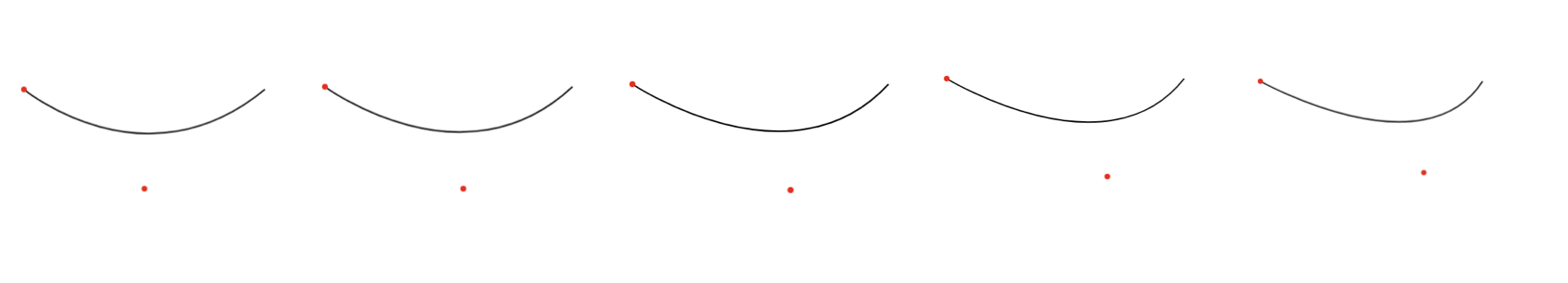Q：只需要指定两组参数，第一组表示控制节点的坐标，第二组表示终点坐标。

```<svg
xmlns="http://www.w3.org/2000/svg"
width="300"
height="300"
>
<path d="M10 80 Q 95 10 180 80" stroke="black" fill="transparent"/>

<circle cx="10" cy="80" r="2" fill="red"/>
<circle cx="95" cy="20" r="2" fill="red"/>
<circle cx="180" cy="80" r="2" fill="red"/>
</svg>```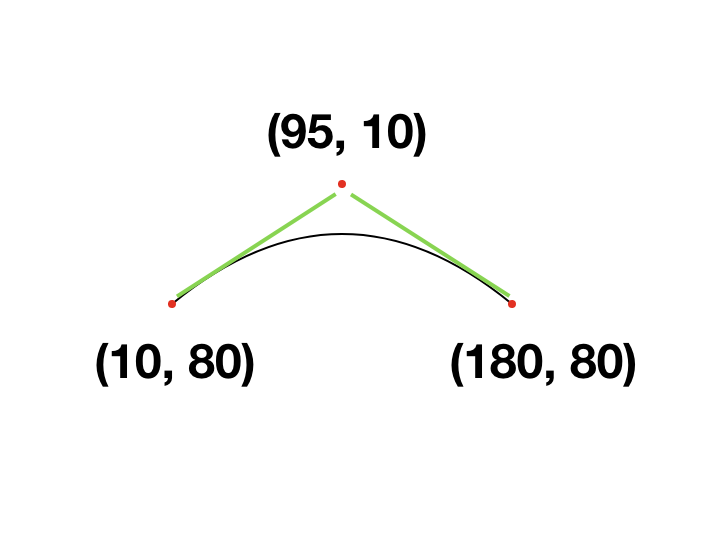T：通过找到前一个控制节点，来推断出一个新的控制点，T指令后面只需要指定一组结束点坐标即可，由于T指令是基于前一个控制点的基础上来生成的，所以T指令之前必须要有Q指令或者其他T指令，否则生成的控制节点就和前一个控制节点就会重合，在画布上看到的就仅仅是一条直线。

``` <svg
xmlns="http://www.w3.org/2000/svg"
width="300"
height="300"
>
<path d="M10 80 Q 52.5 10, 95 80 T 180 80" stroke="black" fill="transparent"/>

<circle cx="10" cy="80" r="2" fill="red"/>
<circle cx="52.5" cy="10" r="2" fill="red"/>
<circle cx="95" cy="80" r="2" fill="red"/>
<circle cx="180" cy="80" r="2" fill="red"/>

</svg>```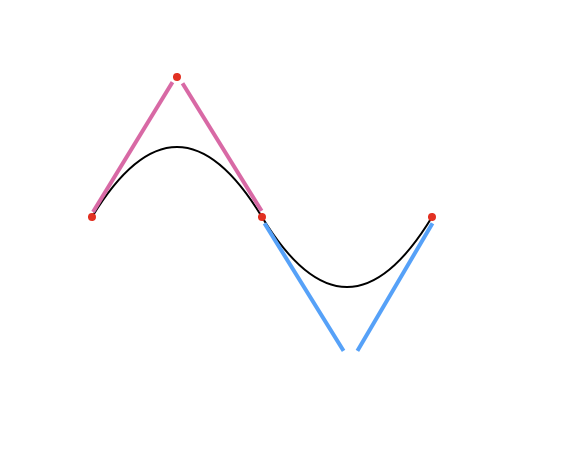• rx：x轴半径
• ry：y轴半径
• x-axis-rotation：弧形的旋转角度
• large-arc-flag：决定弧线是大于180度好事小于180度，0表示小角度弧，1表示大角度弧
• sweep-flag：表示弧线的方向，0表示从起点到终点沿逆时针画弧，1表示从起点到终点沿顺时针画弧
• x：弧形终点的横坐标
• y：弧形终点的纵坐标

``` <?xml version="1.0" standalone="no"?>
<svg width="325px" height="325px" version="1.1" xmlns="http://www.w3.org/2000/svg">
<path d="M80 80
A 45 45, 0, 0, 0, 125 125
L 125 80 Z" fill="green"/>
<path d="M230 80
A 45 45, 0, 1, 0, 275 125
L 275 80 Z" fill="red"/>
<path d="M80 230
A 45 45, 0, 0, 1, 125 275
L 125 230 Z" fill="purple"/>
<path d="M230 230
A 45 45, 0, 1, 1, 275 275
L 275 230 Z" fill="blue"/>
</svg>```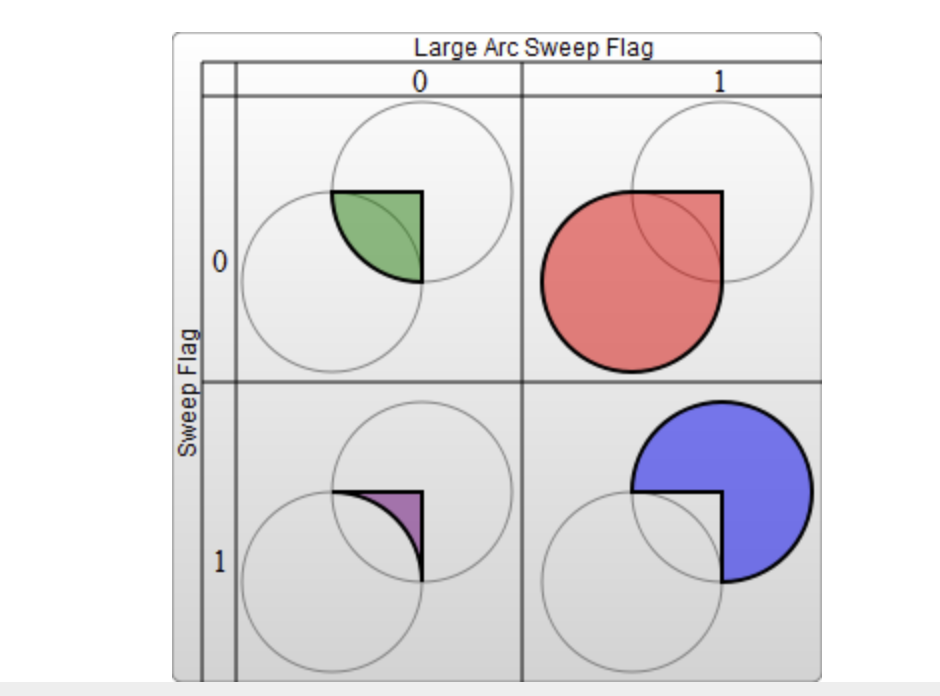``` <svg width="325" height="325" xmlns="http://www.w3.org/2000/svg">
<path d="M80 80
A 45 45, 0, 0, 0, 125 125
L 125 80 Z" fill="green"/>
<path d="M170 80
A 45 45, 0, 0, 1, 125 125
L 125 80 Z" fill="red"/>
<path d="M170 80
A 45 45, 0, 0, 0, 125 35
L 125 80 Z" fill="blue" />
<path d="M80 80
A 45 45, 0, 0, 1, 125 35
L 125 80 Z" fill="pink"/>
</svg>```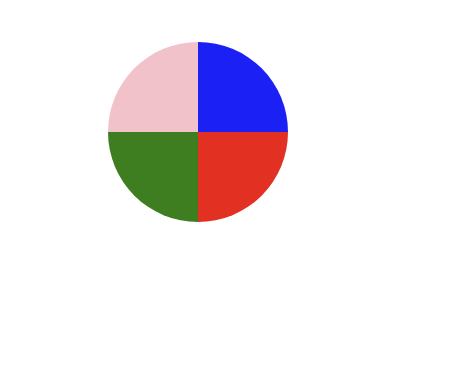MDN SVG